Monday, October 26, 2015

Defining Forward DWT through Recurrences: Conceptual Note 1 on DWT Scale Notation in Ripples in Mathematics

Introduction

This is a conceptual note on Ch. 3, pp. 22-23, in "Ripples in Mathematics" by A. Jensen & A. la Cour-Harbo. I had implemented the 1D and 2D Haar Transforms (my github repo is here) before reading this great and beautiful book. So, on my first reading, I quickly read through the notation on p. 22. However, when I started implementing CDF(2, 2), the issue of notation became important. Below are the modifications that I introduced to the DWT scale notation adopted in Ch 3 to understand CDF(2, 2).  The notation for the forward DWT used in Ch. 3 is given in Fig. 1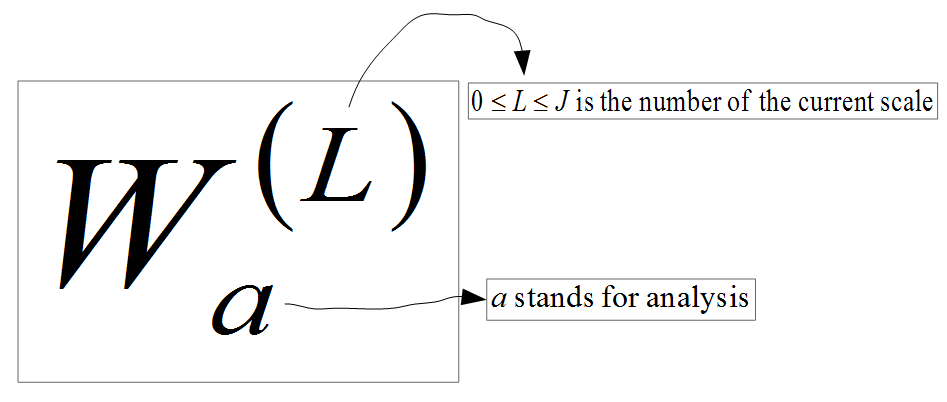Figure 1. Notation for Forward DWT

The forward direct wavelet transform (DWT) is applied to a signal of length 2^J, for J > 0. Each application of the transform is called a scale. The maximum scale value is J.  I take scale 0 to be the original signal. A signal is a sequence of samples, e.g., real numbers. At each scale greater than 0, the signal can be represented as the signal proper, i.e., the scaled signal, and the difference sequence (this term is mine). The difference sequence consists of numbers that allow us to recover the signal at the previous scale and to reason about the signal properties, e.g., how the signal changes from the 1st half to the second half. Fig. 2 shows the notation I used to represent a signal at a specific scale to make sense of the CDF(2, 2) definition on p. 23. This notation differs slightly from the one used in the book and may be helpful to the readers of this document when they implement CDF(2, 2).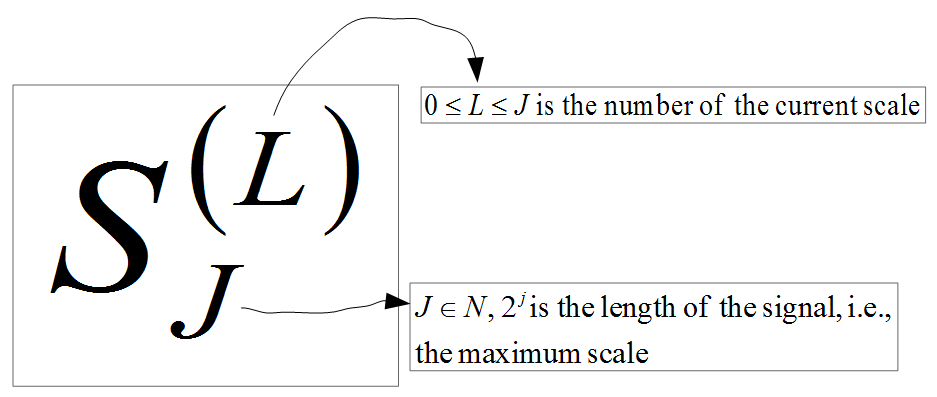Figure 2. Notation for Signal at Scale L
Fig. 3 gives my notation for the i-th sample of a scaled signal. In other words, the symbol defined in Fig. 2 denotes a signal whose individual elements are referenced by symbols defined in Fig. 3.  Note that in Figures 3 and 5, the range for i should be defined as 0 <= i < 2^(J-L), not 0 <= i <= 2^(J-L). I noticed this typo after I published this post.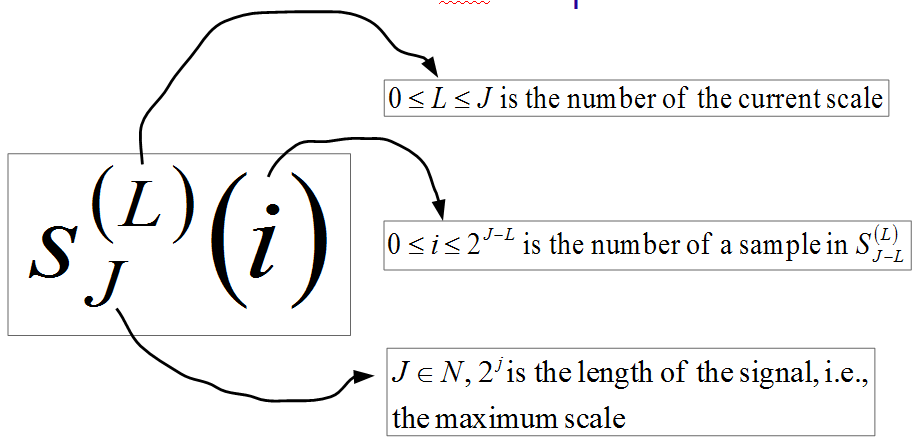Figure 3. Notation for the I-th Sample of the Signal at Scale L
The second component of the DWT at a given scale is the difference sequence. I do not think difference sequence is a standard term. The notation for difference sequences is shown in Fig. 4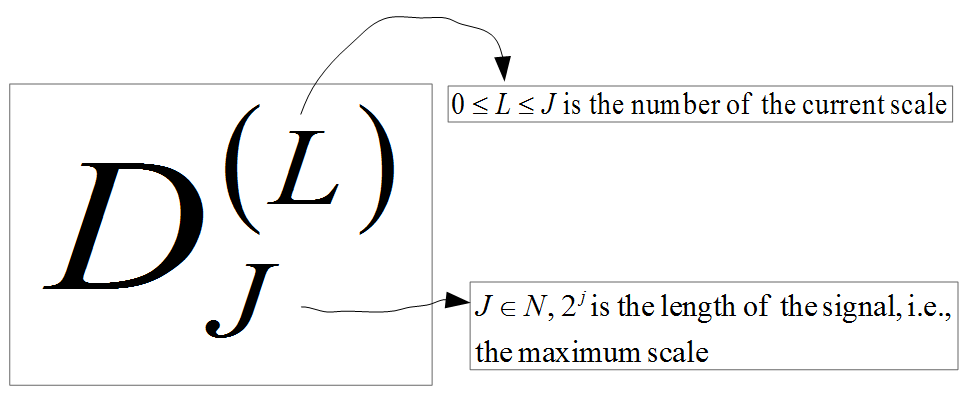Figure 4. Notation for the Difference Sequence at Scale L
Fig. 5 shows the notation used for individual elements of difference sequences.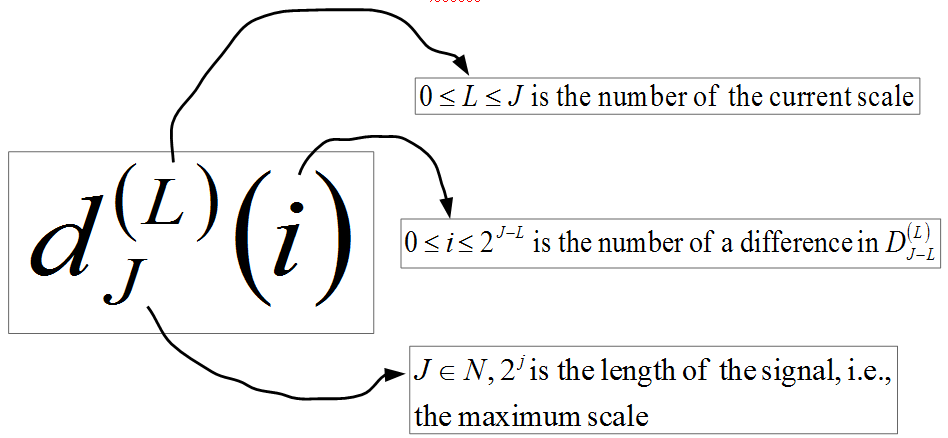Figure 5. Notation for the I-th Difference in the Difference Sequence at Scale L
To denote the DWT of a signal of length 2^J at a scale L, I used the notation given in Fig. 6. T stands for "transform."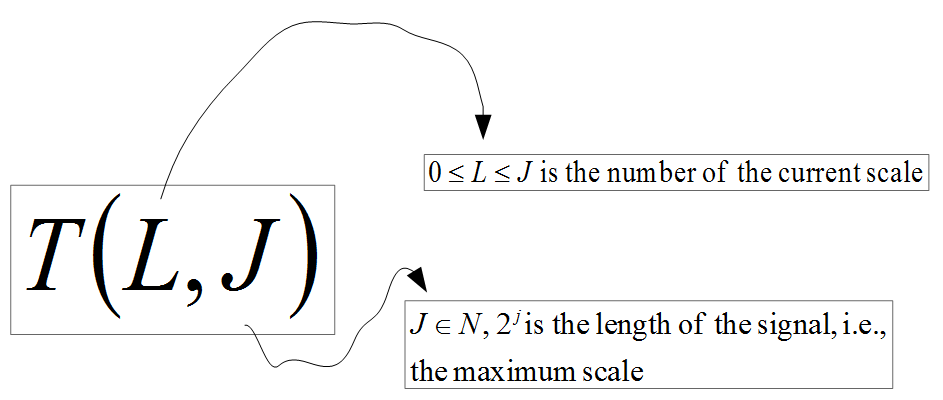Figure 6. Notation for the Direct Wavelet Transform of a Signal of length 2^J at Scale L
Finally, Fig. 7 defines T(L, J) through recurrences.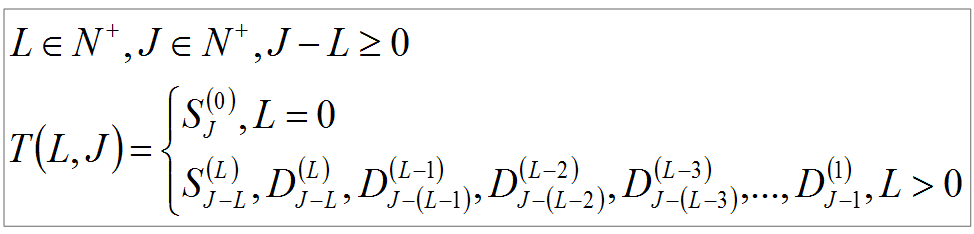Figure 7. Recurrences for T(L, J)

Example 1

Figures 8 - 9 shows the DWT of a signal with 2 samples.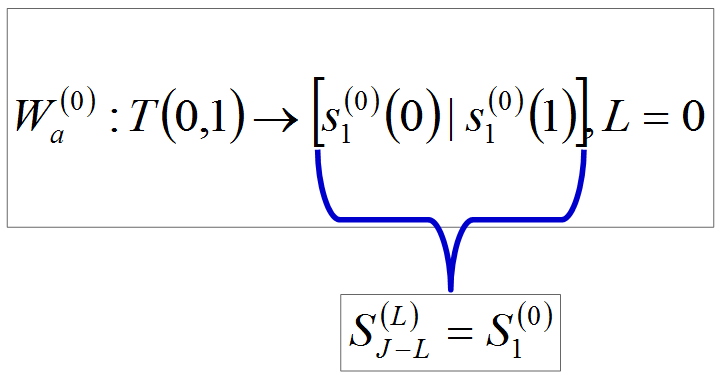Figure 8. T(0, 1)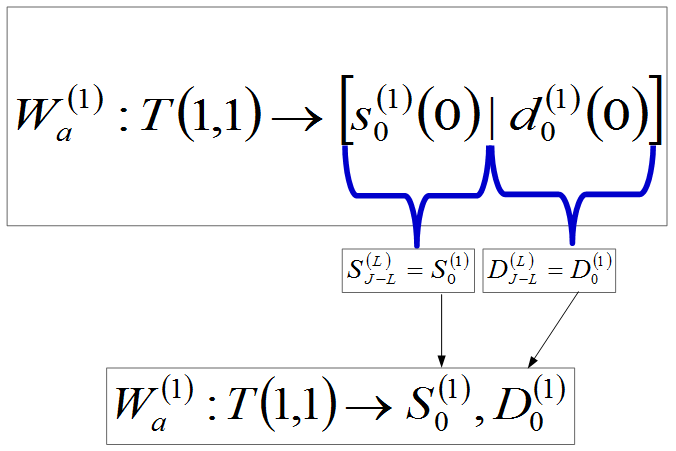Figure 9. T(1, 1)

Example 2

Figures 10 - 12 shows the DWT of a signal with 4 samples.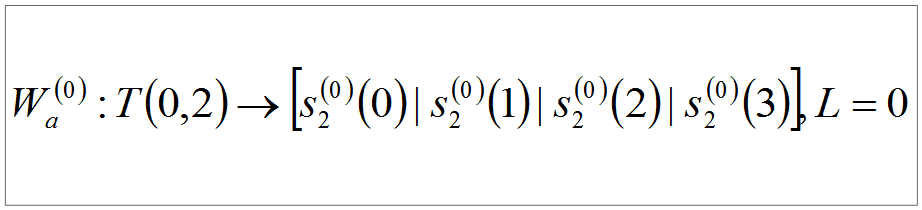Figure 10. T(0, 2)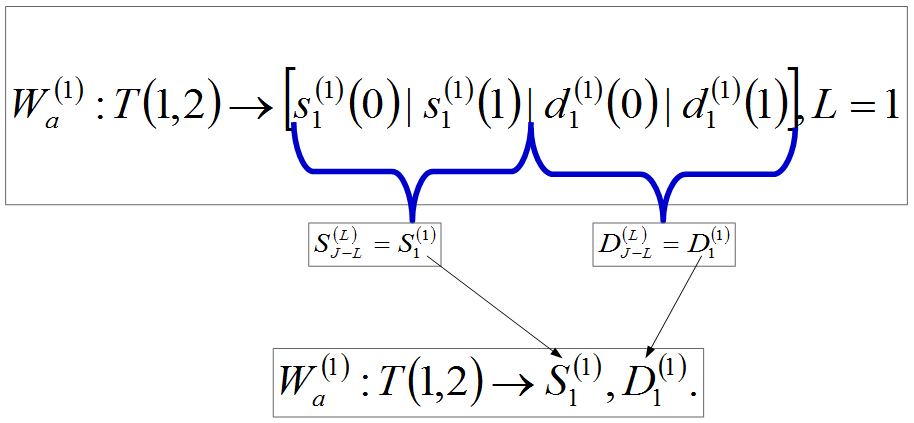Figure 11. T(1, 2)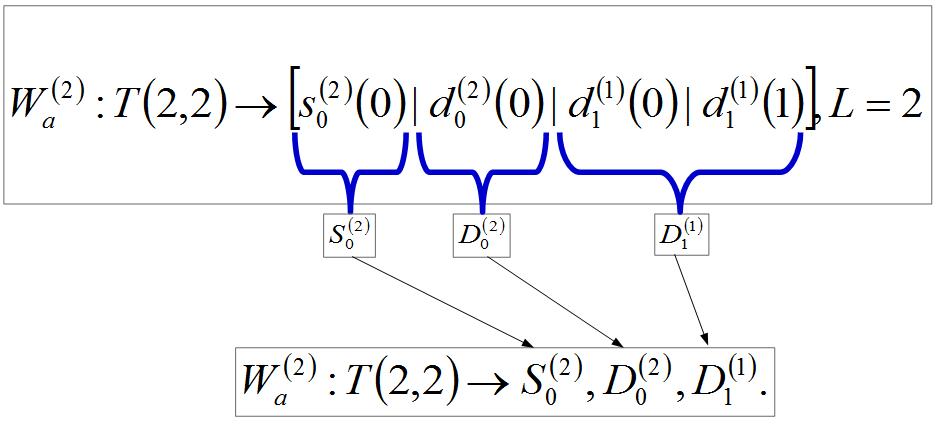Figure 12. T(2, 2)

Example 3

Figures 13 - 16 shows the DWT of a signal with 8 samples.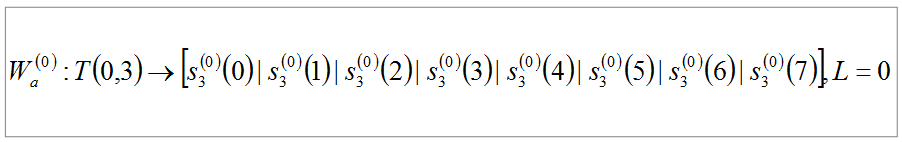Figure 13. T(0, 3)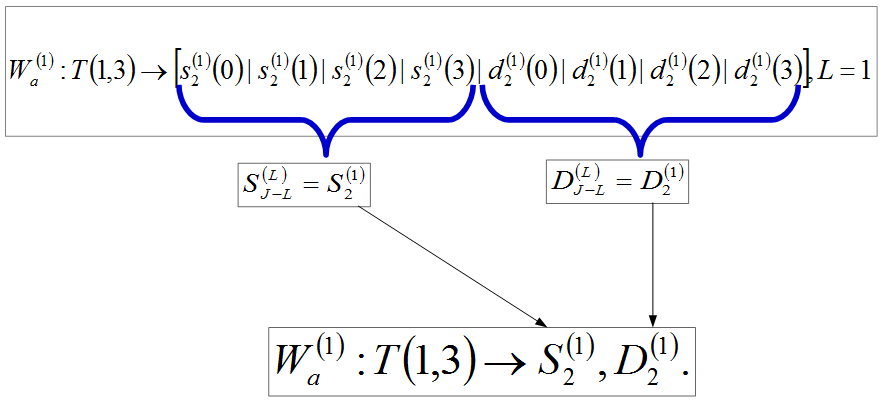Figure 14. T(1, 3)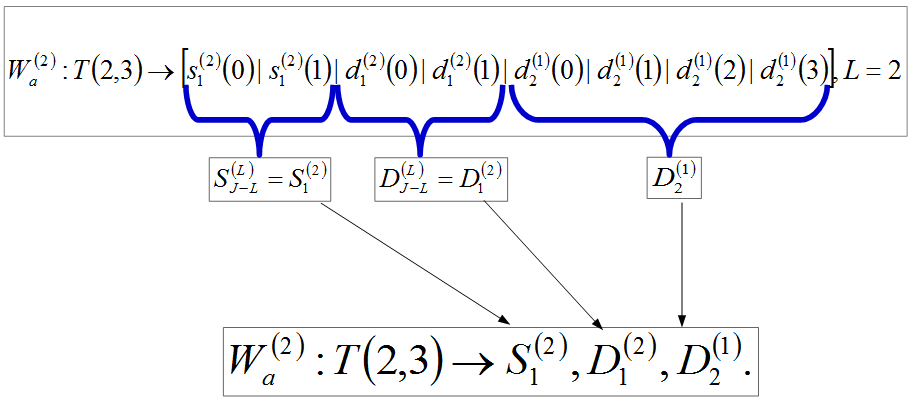Figure 15. T(2, 3)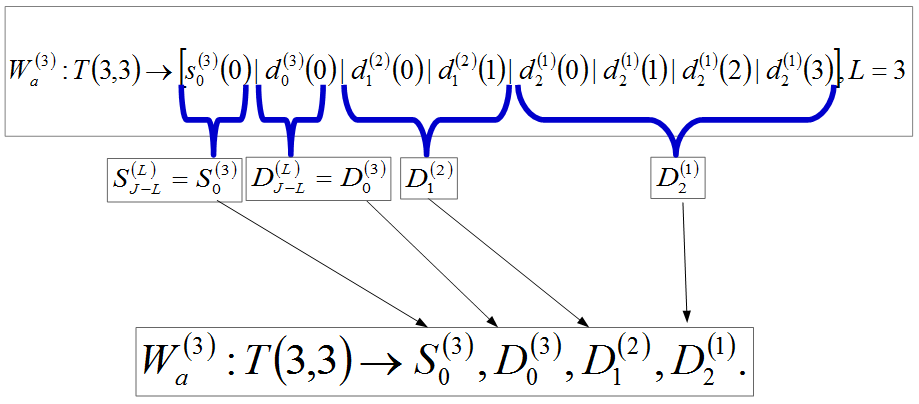Figure 16. T(3, 3)